# Common Core: High School - Geometry : Parallelogram Proofs

## Example Questions

← Previous 1

### Example Question #1 : Parallelogram Proofs

Which of the following is the definition of a parallelogram?

A quadrilateral with four congruent sides and four congruent angles

A quadrilateral with two pairs of opposite parallel sides

A quadrilateral with one pair of parallel sides

A quadrilateral with four right angles

A quadrilateral with two pairs of opposite parallel sides

Explanation:

A parallelogram is a quadrilateral with two pairs of opposite parallel sides such as the following.Opposite sides of a parallelogram are congruent as well as its opposite angles.

### Example Question #1 : Parallelogram Proofs

Which of the following is the definition of a parallelogram?

A quadrilateral with four congruent sides and four congruent angles

A quadrilateral with one pair of parallel sides

A quadrilateral with two pairs of opposite parallel sides

A quadrilateral with four right angles

A quadrilateral with two pairs of opposite parallel sides

Explanation:

A parallelogram is a quadrilateral with two pairs of opposite parallel sides such as the following.Opposite sides of a parallelogram are congruent as well as its opposite angles.

### Example Question #532 : High School: Geometry

Which of the following is the definition of a parallelogram?

A quadrilateral with four right angles

A quadrilateral with four congruent sides and four congruent angles

A quadrilateral with one pair of parallel sides

A quadrilateral with two pairs of opposite parallel sides

A quadrilateral with two pairs of opposite parallel sides

Explanation:

A parallelogram is a quadrilateral with two pairs of opposite parallel sides such as the following.Opposite sides of a parallelogram are congruent as well as its opposite angles.

### Example Question #1 : Parallelogram Proofs

Prove that the following parallelogram has diagonals that prove two congruent triangles.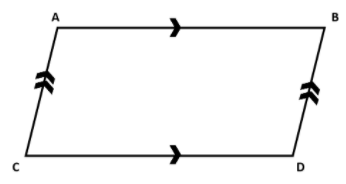Proof: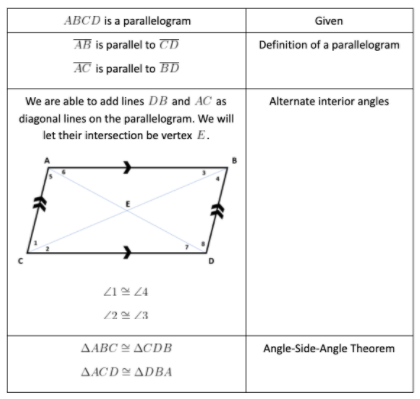Proof: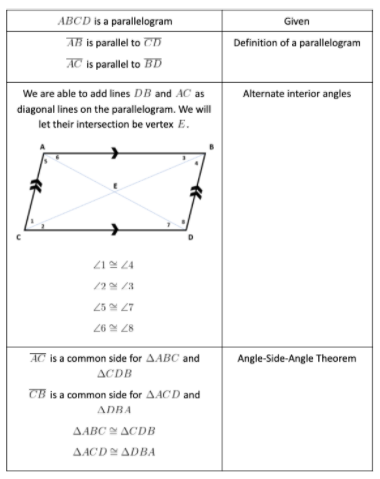Proof: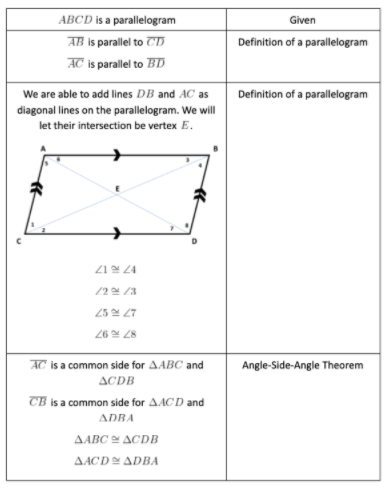Proof:Explanation: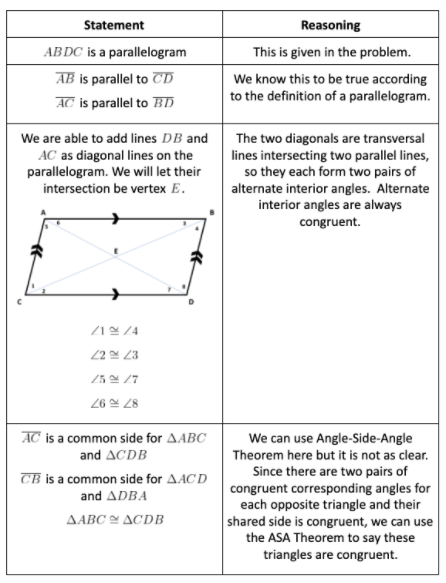### Example Question #4 : Parallelogram Proofs

Prove that the following parallelogram has four pairs of consecutive supplementary angles.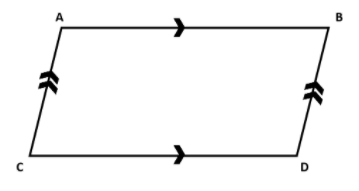Proof: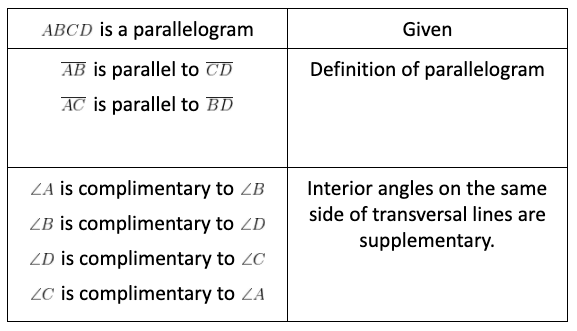Proof: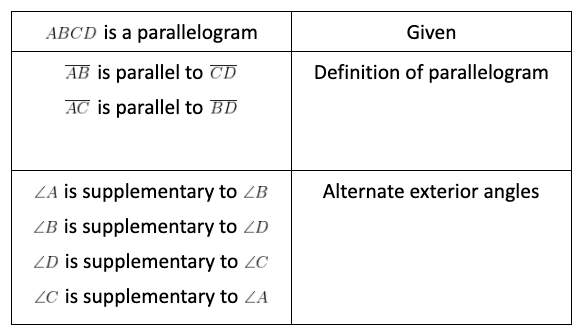Proof: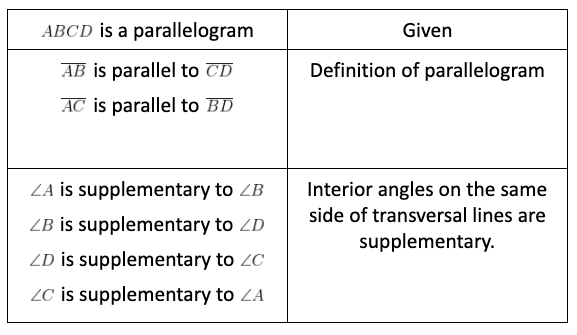Proof:Explanation: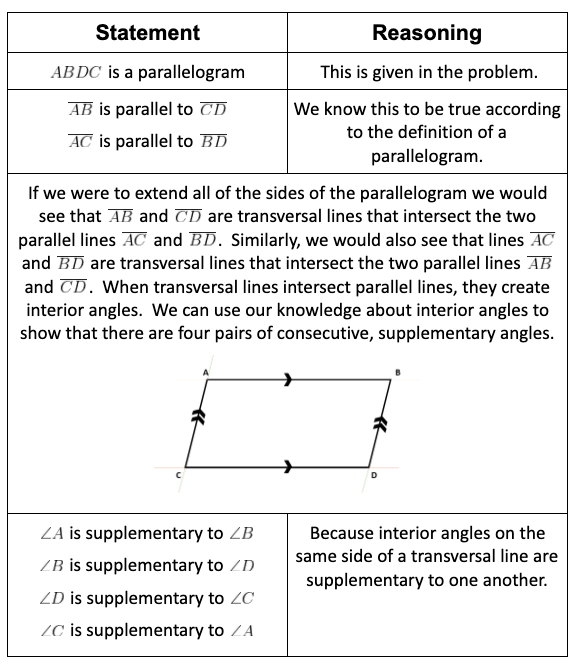Thus, we have shown that this parallelogram has four pairs of consecutive supplementary angles.

### Example Question #11 : Prove Parallelogram Theorems: Ccss.Math.Content.Hsg Co.C.11

Prove the following parallelogram has two pairs of opposite congruent angles.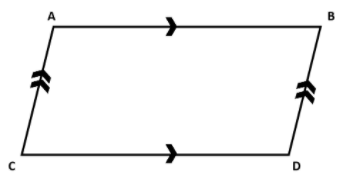Proof: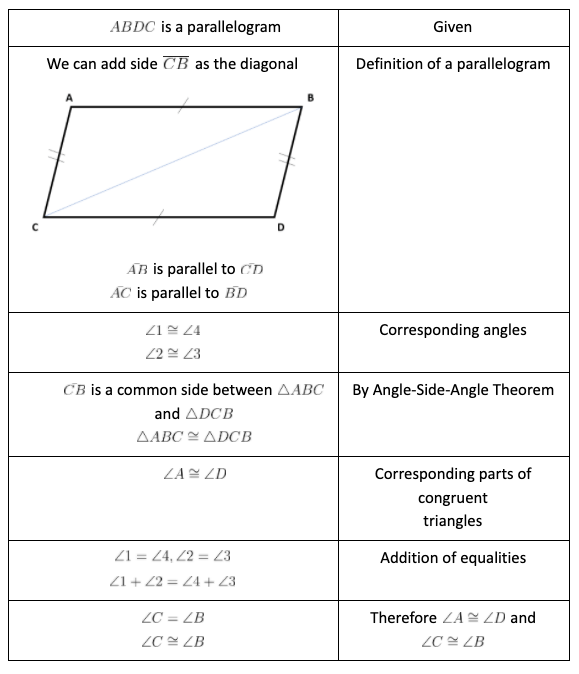Proof: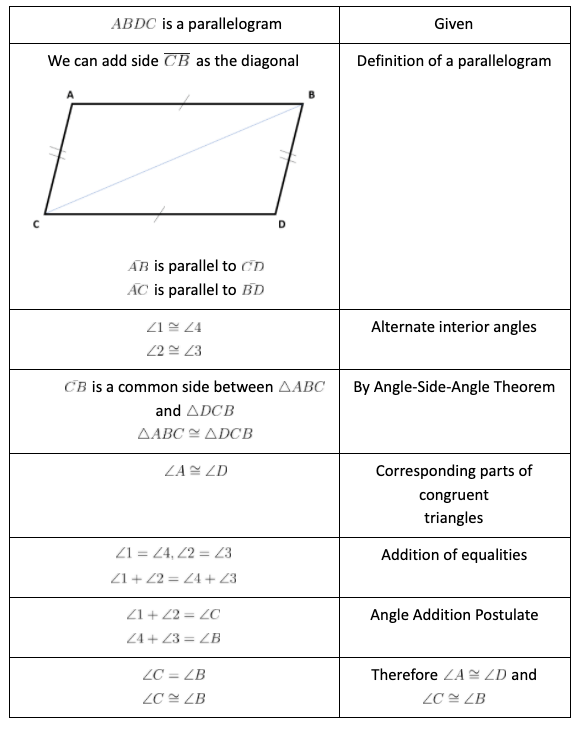Proof: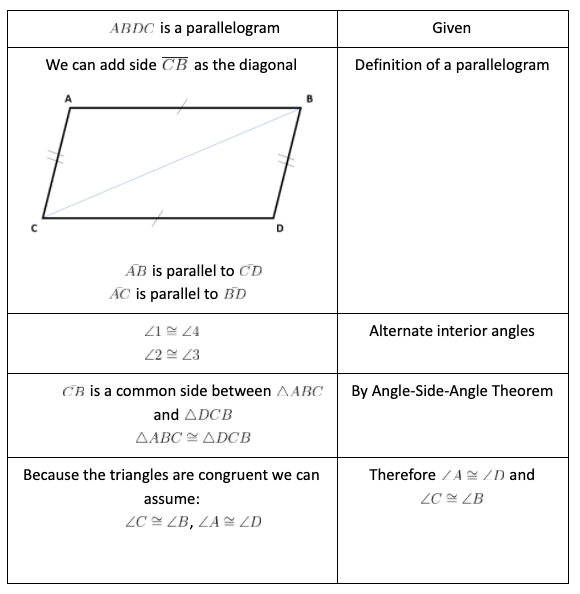Proof:Explanation: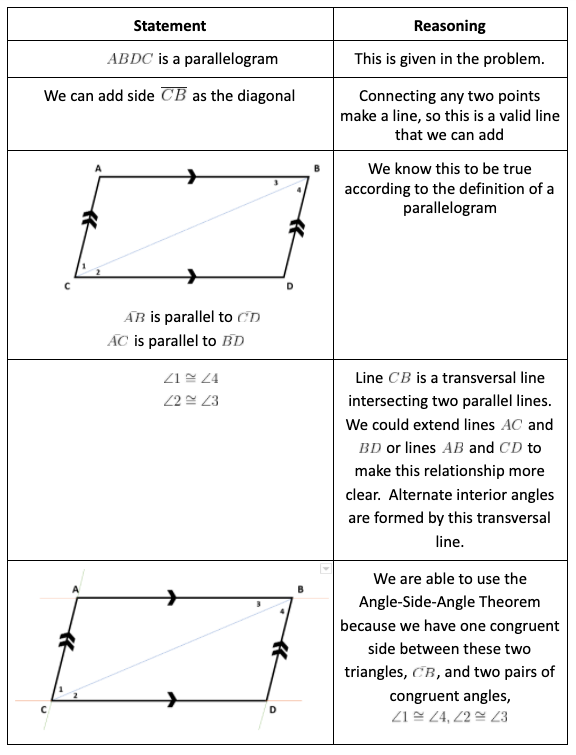### Example Question #491 : High School: Geometry

Prove the following parallelogram has opposite congruent sides.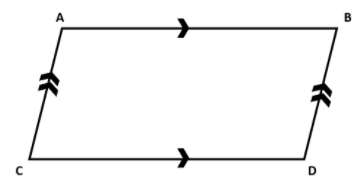Proof: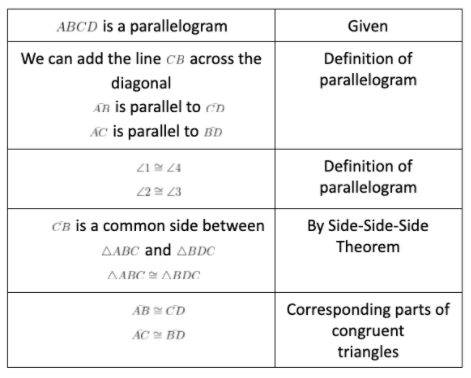Proof: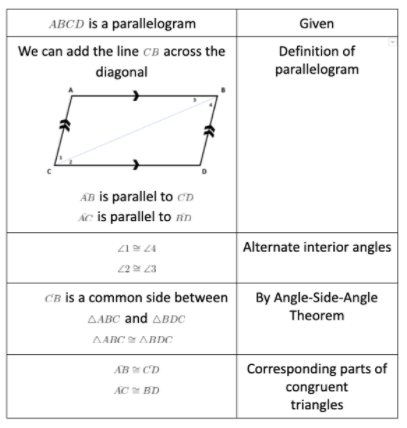Proof: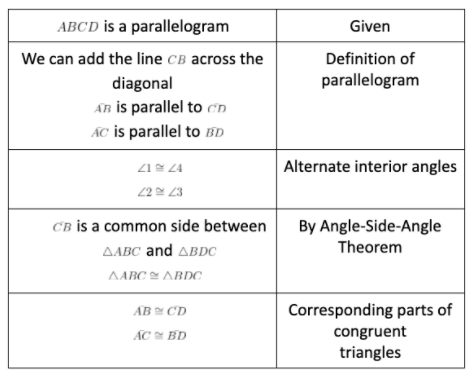Proof:Explanation: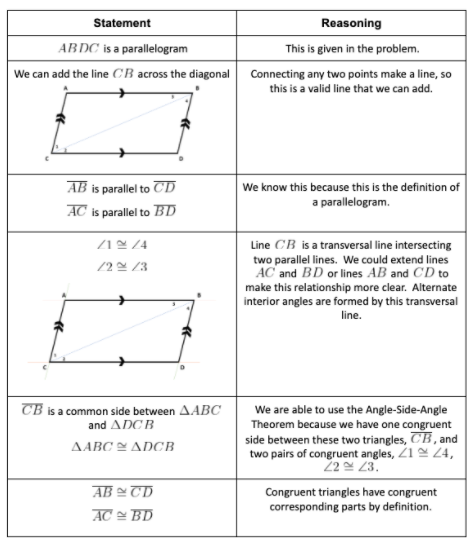Thus, we have proven this parallelogram has congruent opposite sides.

### Example Question #5 : Parallelogram Proofs

Prove that since this quadrilateral has two pairs of opposite congruent sides, it is a parallelogram.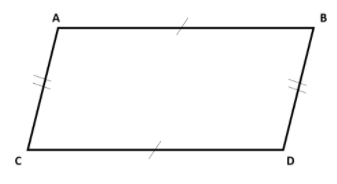Proof: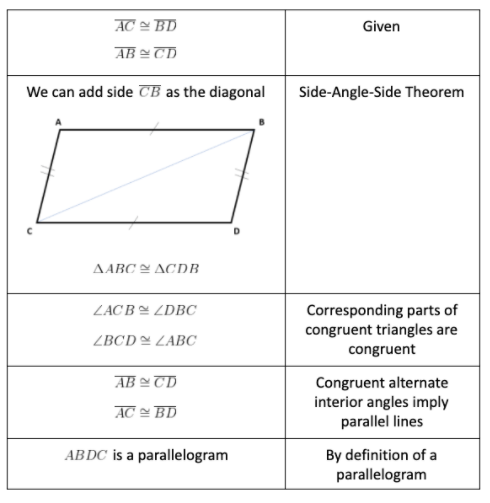Proof: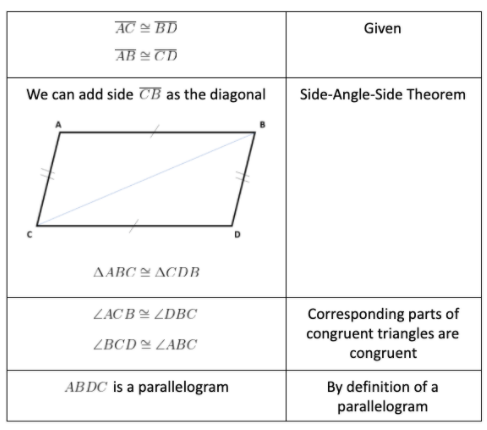Proof: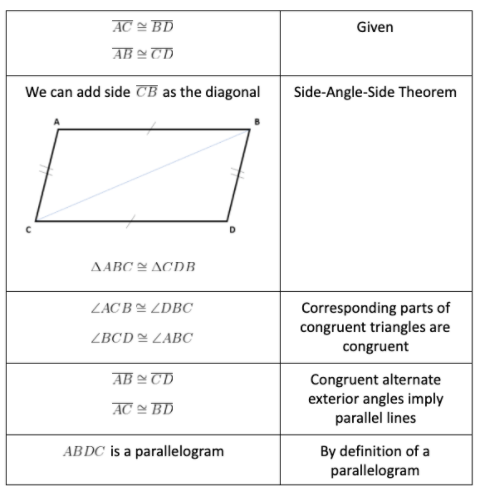Proof:Explanation: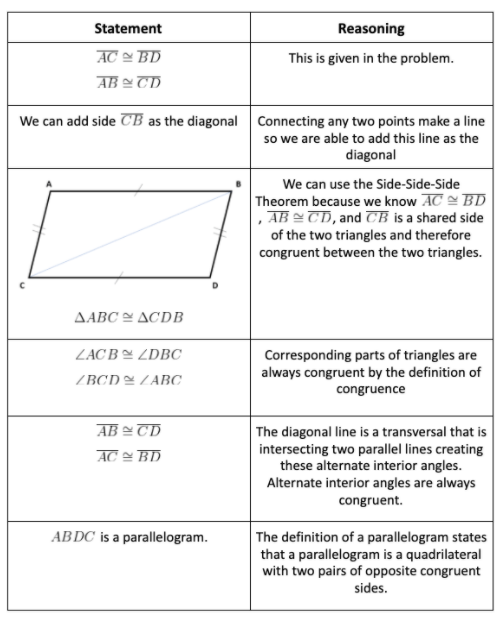Thus, we have shown that if a quadrilateral has two pairs of congruent opposite sides, then it is a parallelogram.

### Example Question #2 : Parallelogram Proofs

True or False: Is the following quadrilateral a parallelogram?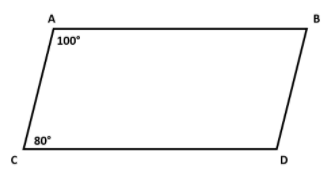True

False

True

Explanation:

This is true because a theorem states that if a quadrilateral has two consecutive angles that are supplementary, then the quadrilateral is a parallelogram.

### Example Question #2 : Parallelogram Proofs

Prove that if the following quadrilateral has a pair of opposite parallel, congruent sides, it is a parallelogram.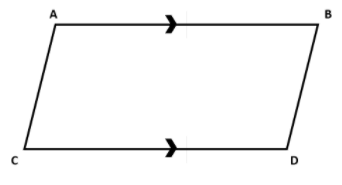Proof: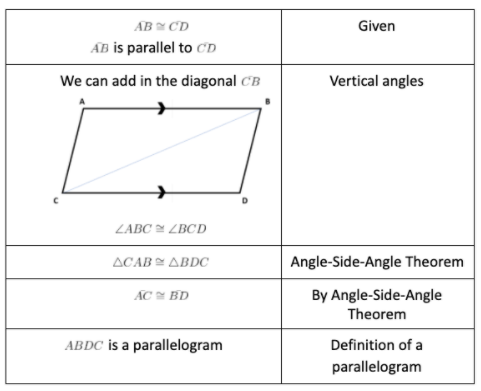Proof: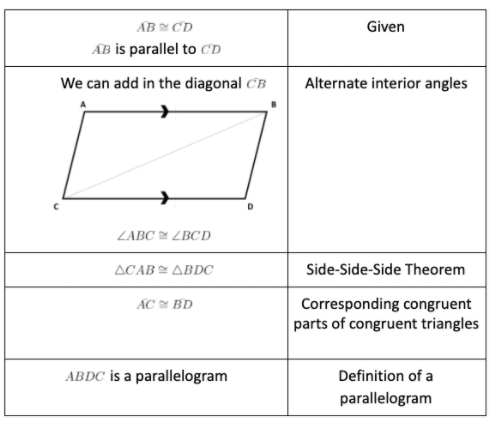Proof: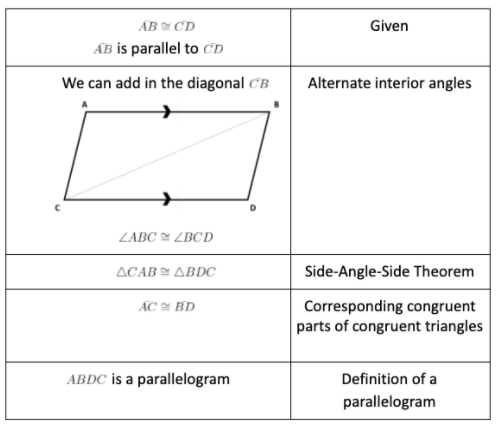Proof:Explanation:

Thus, we have shown that if a quadrilateral has one pair of parallel, congruent opposite sides, then it is a parallelogram.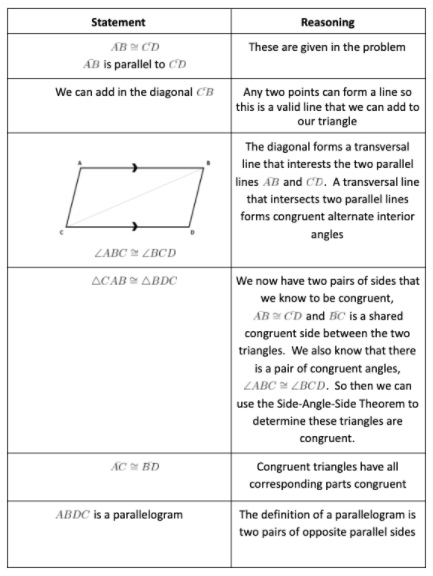← Previous 1

### All Common Core: High School - Geometry Resources